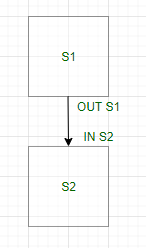GeeksforGeeks App
Open AppBrowser
Continue

# GATE | GATE CS 2021 | Set 2 | Question 48

For a statement S in a program, in the context of liveness analysis, the following sets are defined:

• USE(S) : the set of variables used in S
• IN(S) : the set of variables that are live at the entry of S
• OUT(S) : the set of variables that are live at the exit of S

Consider a basic block that consists of two statements, S1 followed by S2. Which one of the following statements is correct?
(A) OUT(S1) = IN (S2)
(B) OUT (S1) = IN (S1) ∪ USE (S1)
(C) OUT (S1) = IN (S2) ∪ OUT (S2)
(D) OUT (S1) = USE (S1) ∪ IN (S2)

Explanation: